### Wilson Mar

Hello!

Email me Calendar Skype call

Github Stackoverflow Pinterest

# (Statistical Multi-Variate) Regression

How to calculate a formula from multiple variables in Excel, and stistics to evaluate them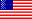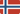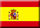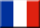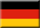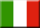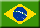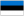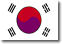### Overview

Here are my notes on how to create a multi-variate Linear Regression formula using Microsoft Excel and Python programs.

NOTE: Content here are my personal opinions, and not intended to represent any employer (past or present). “PROTIP:” here highlight information I haven’t seen elsewhere on the internet because it is hard-won, little-know but significant facts based on my personal research and experience.

## Evaulation of regression fit

“Loss” is how far off actual values are from the estimation function (model).

To define the total loss for a function, we want to examine the length of loss regardless of whether it’s over or under. Thus, we calculate the square of each sample loss so negative values are treated as positive.

Mean square error (MSE) is the average squared loss per example, calculated by summing up all the squared losses for individual examples, then dividing by the number of examples.

Although MSE is commonly-used, it is neither the only practical loss function nor the best loss function for all circumstances.

Which one is best?

• Coefficient of Determination (R2): (aka “R-Squared) is a relative measure of how well the model fits dependent variables. It summarizes the variance between predicted and true being explained by the model. The closer to 1 this value is, the better the model is performing. It does not take into consideration of overfitting problem if it performs poorly with training data. Thus:

MSE, RMSE or MAE are used to compare performance between different regression models:

• Mean Absolute Error (MAE) is an absolute measure of the goodness for the fit. It gives you an absolute number on how much your predicted results deviate from the actual number. The average difference between predicted vs. true values. This value is based on the same units as the label, such as dollars. The lower this value is, the better the model is predicting.

• Root Mean Squared Error (RMSE) is used by Kaggle to assess submissions for its competition. The square root of the mean squared difference between predicted and true values. The result is a metric based on the same unit as the label (dollars). A larger difference When compared to the MAE (above) indicates greater variance in the individual errors (for example, with some errors being very small, while others are large).

To compare models where labels are in different units:

• Relative Absolute Error (RAE): A relative metric between 0 and 1 based on the absolute differences between predicted and true values. The closer to 0 this metric is, the better the model is performing.

• Relative Squared Error (RSE): A relative metric between 0 and 1 based on the square of the differences between predicted and true values. The closer to 0 this metric is, the better the model is performing.

## Obtain Sample Data

We need some sample data to build a linear regression model to provide results and a recommendation.

One is predicting expected profits from a catalog launch by a home-goods manufacturer.

The training set contains historical data where the outcome is already known and included as a column along with the input data.

Outcome values are in the target variable (price).

NOTE: A column in a spreadsheet some call a “data field”.

2. The test dataset (new-diamonds.csv) contains new instances that do not have outcomes (prices) defined.

### Install Excel

This tutorial assumes that you have Microsoft Excel installed.

To create a multi-variate regression in Excel:

This video is from the Udacity class, about a different data set

### Open in Excel

During the installation processes on Windows or MacOS, the operating system is told that suffixes at the end of file names, such as “.csv” and “.xlsx” are opened using Excel.

3. In Finder or double-click on the diamonds.csv file to open it in Excel.

### Activate Analysis Toolpak

4. In Excel, click on the Data tab.

If Data Analysis does not appear on the far right, the Analysis Toolpak needs to be installed:

On Excel 2016, click the Tools menu at the top of the screen to select Excel Add-ins. Check Analysis ToolPak, then OK.

On Excel 2013, click the File menu at the top of the screen to select Properties. Check Analysis ToolPak, then OK.

The above only needs to be done once.

## Data preparation

### Contiguous X columns

Excel needs all variables together, so:

5. Select the letter above the “caret” column to select the whole column.
6. Press Ctrl+X to cut. Click on the column heading to the right of the “price” column.
7. Repeat for the “clarity_ord” and “cut_ord” columns.

### Generate

8. In Excel Data tab, Click on Data Analysis.
9. If you don’t see “Data Analysis”, enable Analysis Toolpack. This only needs to be done once.
10. Select Regression and OK.

Conditions of attributes are in the predictor variables, also called dependent variables or (in Excel), Y Range.

11. Click the red arrow icon for Y Range.
12. Check “Labels”
13. Click in row 1 “price”. Press Command-shift-down to select the whole column

\$H\$1:\$H\$50001

14. Click the icon to collapse the pop-up.
15. Press Enter.

\$I\$1:\$K\$50001

16. Click the icon to Output range.
17. Cick cell N1, which leaves two blank columns.
18. Press Enter to accept it.

19. Click OK.

20. Variables with P-values (Prediction-values) beyond .15 should not be used.

Regression equation models enable predictions to be made based on attibute values.

###

21. Click on the blank cell to the left of “intercept”, type = and click the Coefficient value of intercept.
22. Two columns to the left of “clarity_ord”, type the input data you want an estimate for.
23. Repeat this for each input.
24. Click on the blank cell to the left of “intercept”, type = and click the Coefficient value of intercept.
25. Add a =sum() of all cells above it.

26. TODO: COMPLETE THIS

R Square is the percent of variance in Y that can be explained by all the X variables. 1.0 is perfect. 0.75 is the threshold for not.

Standard Error is the error of prediction expressed in the value range of Y.

## Labs and classes

There are several classes that teach this topic:

If you have an O’Reilly subscription: https://learning.oreilly.com/scenarios/linear-regression-with/9781492098386/

Datacamp Video course Multiple and Logistic Regression on-line class by Ben Baumer, Assistant Professor at Smith College uses a database of Italian restaurants in New York City to explore the relationship between price and the quality of food, service, and decor. Learn about logistic regression for an arbitrary number of input variables. Covered are model and predict both numeric and categorical outcomes. You’ll also learn how to fit, visualize, interpret, and compare models. Learn about the class of linear models called “parallel slopes models.” Learn to compare models so that you can select the best one. Add two, three, and even more numeric explanatory variables to a linear model.

The nice thing about datacamp’s videos is that one can highlight and copy text from videos, such as this:

```ggplot(data = mpg_manuals, aes(x = factor(year), y = hwy)) +
geom_boxplot()
```

This 10-hour course on YouTube about Regression Analysis helps you understand relationships in your data. Learn concepts that underpin many machine learning algorithms, such as Linear Regression, Polynomial Regression, Feature Engineering, and more. Code several Python projects.

https://hbr.org/2015/11/a-refresher-on-regression-analysis A Refresher on Regression Analysis by Amy Gallo

https://www.wikiwand.com/en/Linear_regression